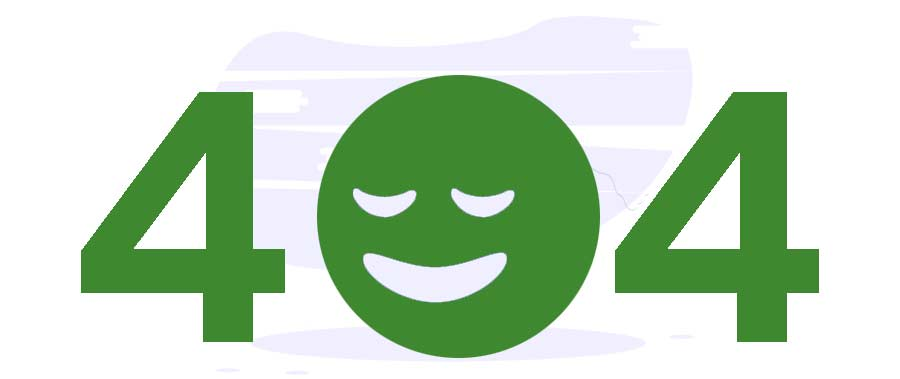# What are Assignment Operators in JavaScript?

Site Under Maintenance - Tutorialspoint# Error. We can’t find the page you’re looking for.

Sorry for the inconvenience. Go to our homepage or check out our Latest Courses, eBooks, Prime Packs...

Back to Home

Using Assignment Operators, you can assign a value to a variable. JavaScript supports the following assignment operators −

Sr.No
Operator and Description
1
= (Simple Assignment )
Assigns values from the right side operand to the left side operand
Ex: C = A + B will assign the value of A + B into C
2
+= (Add and Assignment)
It adds the right operand to the left operand and assigns the result to the left operand.
Ex: C += A is equivalent to C = C + A
3
−= (Subtract and Assignment)
It subtracts the right operand from the left operand and assigns the result to the left operand.
Ex: C -= A is equivalent to C = C – A
4
*= (Multiply and Assignment)
It multiplies the right operand with the left operand and assigns the result to the left operand.
Ex: C *= A is equivalent to C = C * A
5
/= (Divide and Assignment)
It divides the left operand with the right operand and assigns the result to the left operand.
Ex: C /= A is equivalent to C = C / A
6
%= (Modules and Assignment)
It takes the modulus using two operands and assigns the result to the left operand.
Ex: C %= A is equivalent to C = C % A

## Example

Try the following code to implement assignment operator in JavaScript −

Live Demo

<html>
<body>
<script>
<!--
var a = 33;
var b = 10;
var linebreak = "<br />";
document.write("Value of a => (a = b) => ");
result = (a = b);
document.write(result);
document.write(linebreak);
document.write("Value of a => (a += b) => ");
result = (a += b);
document.write(result);
document.write(linebreak);
document.write("Value of a => (a -= b) => ");
result = (a -= b);
document.write(result);
document.write(linebreak);
document.write("Value of a => (a *= b) => ");
result = (a *= b);
document.write(result);
document.write(linebreak);
document.write("Value of a => (a /= b) => ");
result = (a /= b);
document.write(result);
document.write(linebreak);
document.write("Value of a => (a %= b) => ");
result = (a %= b);
document.write(result);
document.write(linebreak);
//-->
</script>
<p>Set the variables to different values and different operators and then try...</p>
</body>
</html>